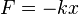## A General Guide to Harmonic Analysis and Beyond

Thanks to Clifford of Asymptotia for the Link too, and from Good Vibration

Some of us do look toward these analogies as signs of Complexity science, so as to apply this thinking to the life they lead. How such implementations allow them to look at this life very differently.

***

Each time the operator tuned to a new frequency, the wave was very simple and repetitive, just as above. This wave can be expressed by only two qualities: frequency and amplitude, and yet each new frequency created a new and many times surprisingly different result than it’s neighbor.

This phenomenon is analogous to all aspects of Complexity Science. And just like the simple rules set by the speaker in the video, an economy can achieve surprisingly complex results with simple rule sets.Harmonic Science

***

In classical mechanics, a harmonic oscillator is a system that, when displaced from its equilibrium position, experiences a restoring force, F, proportional to the displacement, x:$F = -k x \,$

where k is a positive constant.

If F is the only force acting on the system, the system is called a simple harmonic oscillator, and it undergoes simple harmonic motion: sinusoidal oscillations about the equilibrium point, with a constant amplitude and a constant frequency (which does not depend on the amplitude).
If a frictional force (damping) proportional to the velocity is also present, the harmonic oscillator is described as a damped oscillator. Depending on the friction coefficient, the system can:

• Oscillate with a frequency smaller than in the non-damped case, and an amplitude decreasing with time (underdamped oscillator).
• Decay exponentially to the equilibrium position, without oscillations (overdamped oscillator).

If an external time dependent force is present, the harmonic oscillator is described as a driven oscillator.

Mechanical examples include pendula (with small angles of displacement), masses connected to springs, and acoustical systems. Other analogous systems include electrical harmonic oscillators such as RLC circuits. The harmonic oscillator model is very important in physics, because any mass subject to a force in stable equilibrium acts as a harmonic oscillator for small vibrations. Harmonic oscillators occur widely in nature and are exploited in many manmade devices, such as clocks and radio circuits. They are the source of virtually all sinusoidal vibrations and waves.

Simple Harmonic Motion Frequency

The frequency of simple harmonic motion like a mass on a spring is determined by the mass m and the stiffness of the spring expressed in terms of a spring constant k

The Landscape “avant la lettre” by A.N. Schellekens

The lowest harmonics correspond to the particles of the Standard Model, plus perhaps a few new particles. The higher harmonics correspond to an infinite series of particles that we can never observe, unless we can build a Planck Energy accelerator

***